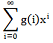# GATE | GATE-CS-2005 | Question 50

Let G(x) = 1/(1 – x)2 =, where | x | < 1. What is g(i) ?
(A) i
(B) i+1
(C) 2i
(D) 2i

Explanation: B is the correct option. Let us put values

```
S = 1 + 2x + 3x2 + 4x3 + ..........
Sx =    x  + 2x2 + 3x3 + ..........
S - Sx = 1 + x + x2 + x3 + ....
S - Sx = 1/(1 - x) [sum of infinite GP series with ratio < 1 is a/(1-r)]
S = 1/(1 - x)2 ```

My Personal Notes arrow_drop_up

Article Tags :

Be the First to upvote.

Please write to us at contribute@geeksforgeeks.org to report any issue with the above content.